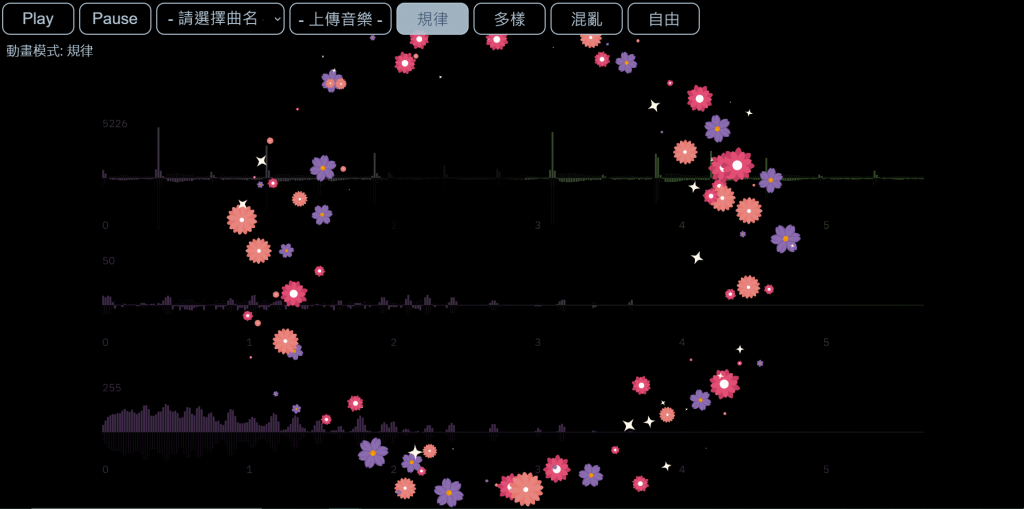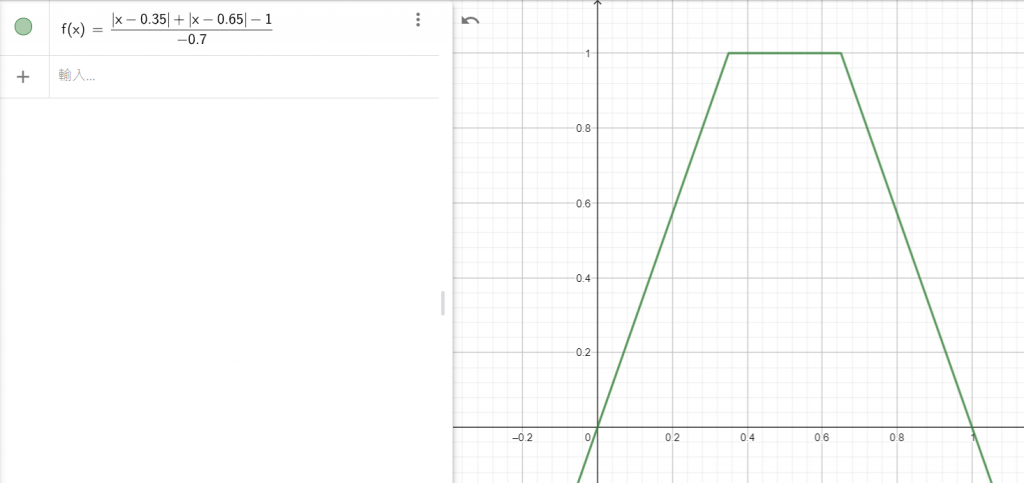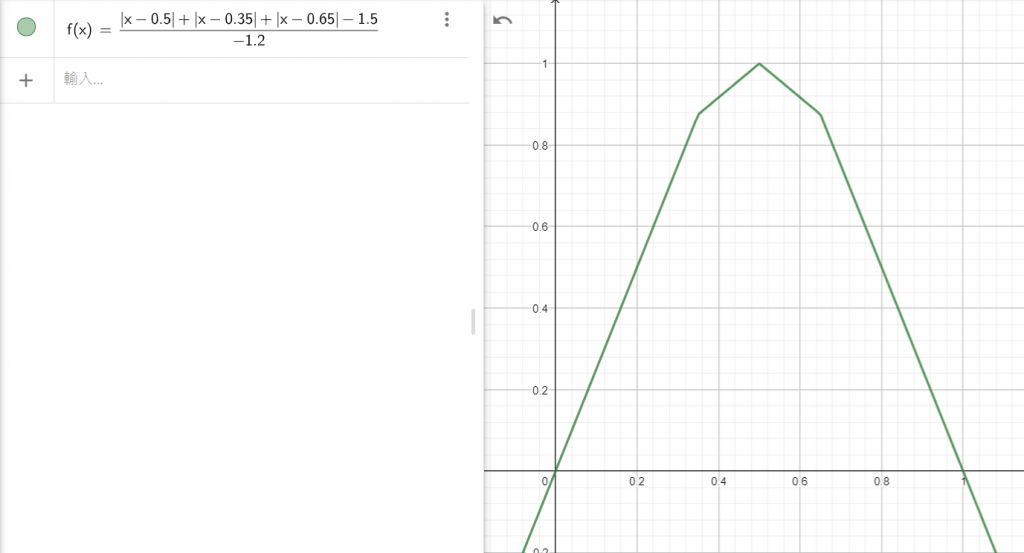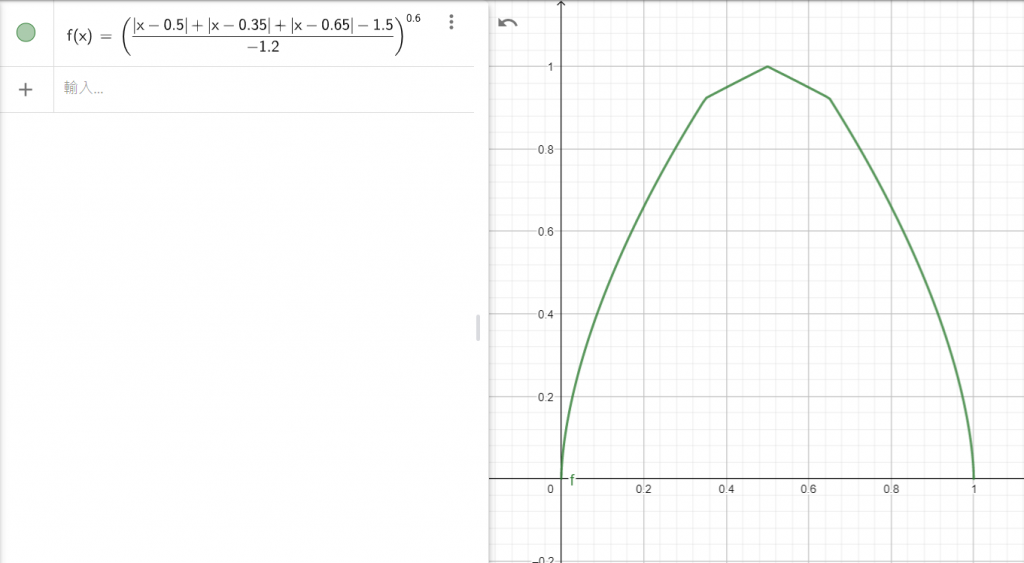#DAY 22
0
Modern Web

## Chapter4 - Canvas背景動畫（III）風中的花朵 今天再加碼讓動畫更加自然的方法

### 一樣先上圖!https://jerry-the-potato.github.io/Chapter4-demo3/

## Staring（因為像星星一樣繞行）

``````switch(animeName){
case 'Falling':
this.beginX = Math.random() * WIDTH;
this.beginY = Math.random() * HEIGHT;
break;
case 'Floating':
this.beginX = Math.random() * WIDTH;
this.beginY = Math.random() * 0.1 * HEIGHT + 0.95 * HEIGHT;
break;
case 'Staring':
this.beginX = Math.random() * 0.1 * WIDTH +  0.45 * WIDTH;
this.beginY = Math.random() * 0.1 * HEIGHT + 0.45 * HEIGHT;
break;
}
``````

``````let lT = this.lifeTime;
let revolveNow = this.revolveTheta + this.revolveOmega * dT / lT;
let A = Math.sin(revolveNow);
let B = Math.cos(revolveNow);
``````

``````let radius = 0.3 * (WIDTH + HEIGHT);
this.pointX = this.beginX + radius * this.scaleX * (this.period * 0.5 * B - 0 * (dT / lT));
this.pointY = this.beginY - radius * this.scaleY * (this.period * 0.5 * A - 0 * (dT / lT));
``````

### 請大家想想看...

（當初第三章就有偷偷放一個其實不是demo的上來，裡面就有這個效果唷！）

## 來聊聊圖案大小的變化### 數線的距離公式

`Math.abs(t - t1) + Math.abs(t - t2) + Math.abs(t - t3);`

t表示輸入的值，我們設定為`dT/lT`，是昨天提到的一次性週期，從動畫開始0-1動畫結束，這邊我們分別設置對稱的三個點(0.35, 0.5, 0.65)，使得公式成為：

`Math.abs(t - 0.35) + Math.abs(t - 0.5) + Math.abs(t - 0.65);`

``````let maxT = 0.5; // 預期圖案在此時最大 max
let minT = 0;   // 預期圖案在此時最小 min
let t1 = 0.35;
let t2 = 0.5;
let t3 = 0.65;
let maxD = Math.abs(maxT - t1) + Math.abs(maxT - t2) + Math.abs(maxT - t3);
let minD = Math.abs(minT - t1) + Math.abs(minT - t2) + Math.abs(minT - t3);
``````

### 映射

``````let dist = Math.abs(dT/lT - t1) + Math.abs(dT/lT - t2) + Math.abs(dT/lT - t3);
let transSize = (dist - minD) / (maxD - minD);
``````

1. 將其先減去最小值，使得dist介於0到(maxD-minD)之間
2. 然後再除以(maxD-minD)，就能使其介於0到1之間

1. 將其先減去1.5(midD)，使距離介於0到-1.2之間
2. 然後除以-1.2(maxD-minD)，使距離介於0到1之間

### 包成函式

``````let Distance = function(t, t1 = 0.35, t2 = 0.5 ,t3 = 0.65){
if(!isNaN(t))
return Math.abs(t - t1) + Math.abs(t - t2) + Math.abs(t - t3);
}
``````

``````animeObject.prototype.GetSize = function(dT, lT){
let maxD = Distance(0.5);
let minD = Distance(0);
let distance = Distance(dT/lT);
let transSize = (distance - minD) / (maxD - minD);
return transSize;
}
animeObject.prototype.Staring = function(dT){
// ......
// 以上省略
const popSize = 0.1;
this.sizeNow = WIDTH * this.size * (popSize + (1 - popSize) * this.GetSize(dT, lT));
}
``````

popSize是初始的參數0.1f(x)=((abs(x-0.5)+abs(x-0.35)+abs(x-0.65)-1.5)/(-1.2))

`return Math.pow(transSize, 0.6);`### 最後還是放一下這三篇動畫的程式碼吧！